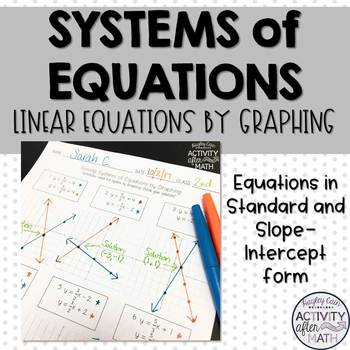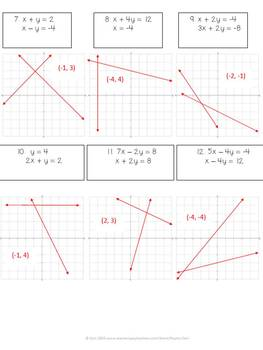Solving Systems of Equations by Graphing Practice Worksheet8th - 9th
Subjects
Standards
Resource Type
Formats Included
• PDF
Pages
9 pages

Description

Students will practice graphing systems of equations written in both slope-intercept form and standard form. All lines are linear equations.

This resource includes:

• Worksheet with 12 problems

*6 problems with equations in Slope-Intercept Form

*6 problems with equations in Standard Form

*Graphs included for each problem

Options for using this resource:

This is a quick way to assess your students knowledge of graphing linear equations and solving systems of linear equations. It can be used as a formative assessment or as a quiz/summative assessment. This is an easy print-and-go and also would be an easy activity to include in your substitute plans.

You might also be interested in these other resources:

Systems of Equations Task Cards with QR Codes

Systems of Equations Coloring Activity

Systems of Equations by Graphing Partner Activity

Wonder Coordinate Graphing Picture

States Coordinate Graphing BUNDLE

Math Movie Questions to accompany Moana

Common Core Aligned

CCSS.MATH.CONTENT.8.EE.C.8.A Understand that solutions to a system of two linear equations in two variables correspond to points of intersection of their graphs, because points of intersection satisfy both equations simultaneously.

CCSS.MATH.CONTENT.HSA.REI.C.6 Solve systems of linear equations exactly and approximately (e.g., with graphs), focusing on pairs of linear equations in two variables.

Don't forget that leaving feedback earns you points toward FREE TPT purchases

activityaftermath@gmail.com

Let's be Social

Check out my Blog

Total Pages
9 pages
Included
Teaching Duration
N/A
Report this Resource to TpT
Reported resources will be reviewed by our team. Report this resource to let us know if this resource violates TpT’s content guidelines.

Standards

to see state-specific standards (only available in the US).
Solve systems of linear equations exactly and approximately (e.g., with graphs), focusing on pairs of linear equations in two variables.
Understand that solutions to a system of two linear equations in two variables correspond to points of intersection of their graphs, because points of intersection satisfy both equations simultaneously.